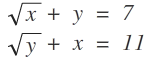# Equation in 2 variables

Algebra Level 1You think you rock at linear equation? Gimme the solution NOT using hit and trial method! Solve for the values of "x" and "y" and enter $x+y$

Note: These are not linear equations.

×